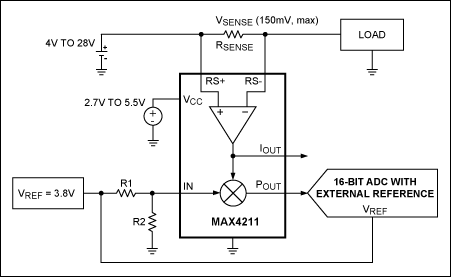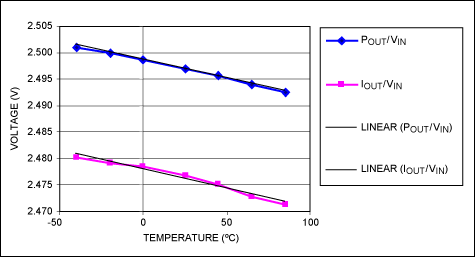# Load-Power Monitor Improves High-Side Current Measurements

### Abstract

Combining a high-side current-sense amplifier with an analog voltage multiplier, MAX4211 shown can easily measure the power dissipated in a load. One multiplier input connects to the load voltage, and the other to an internal analog of the load current—that is, a proportional voltage produced by the internal current-sense amplifier. The multiplier output (VLIL) is then a voltage proportional to load power.

A similar version of this article appeared in the August 1, 2008 issue of Power Electronics Technology magazine.

An IC that combines a high-side current-sense amplifier with an analog voltage multiplier (MAX4211) can easily measure the power dissipated in a load. One multiplier input connects to the load voltage, and the other to an internal analog of the load current—i.e., a proportional voltage produced by the internal current-sense amplifier. The multiplier output (VLIL) is then a voltage proportional to load power.

The internal multiplier can also enable extra precision in high-side current measurements, for applications in which the current signal is digitized by an A-D converter. Whether the ADC's voltage reference is internal or external to the ADC, the accuracy of the digitized load-current measurement depends strongly on the accuracy and stability of that reference.

To minimize this dependency on voltage-reference accuracy, connect the multiplier's external input to the reference voltage via a resistive divider (Figure 1). The current measurement is then ratiometric: any error or drift in the reference voltage has a proportional effect on the ADC's input, and thereby achieves a first-order cancellation of full-scale error caused by the reference voltage. The circuit shown can measure battery charge and discharge currents in a wide range of applications, and it works equally well with a voltage reference internal to the ADC, driving the R1-R2 divider.The IC's multiplier output (POUT) feeds a 16-bit ADC whose input voltage range is 0V to VREF. VREF, provided here by an external voltage regulator, should be between 1.2V and 3.8V (3.8V in this case). The multiplier input must be limited to a range of 0V to 1V, which is accomplished by dividing the 3.8V reference voltage with the R1/R2 resistor divider. Assuming R2 = 1kΩ and R1 = 2.8kΩ, then VIN = 1V. The IC has a gain of 25 between VSENSE and IOUT, and a sense-voltage range (VSENSE) of 0V to 150mV, which produces (at both POUT and IOUT) an output in the range 0V to 3.75V.

Thus, the use of POUT (instead of IOUT) confers an advantage: the signal fed to the ADC, which is proportional to current in the load, is scaled by VREF. The following equation relates the POUT/VREF ratio to ILOAD, RSENSE, and the values of R1 and R2:

POUT/VREF = ILOAD × RSENSE × 25 × VREF × R2/(R1 + R2)/VREF = ILOAD × RSENSE × 25 × R2/(R1 + R2)

Note that the ratio of ADC input to ADC full-scale (POUT/VREF) does not depend on the accuracy of VREF.

Overall accuracy of the current measurement depends on many factors: resistor tolerance, amplifier gain error, voltage offset and bias current, reference voltage accuracy, ADC errors, and drift versus temperature for all the above. This circuit improves accuracy by eliminating only one of these causes—the reference voltage inaccuracy. VREF is affected by at least three sources of error:

• Initial dc error as a percentage of the nominal value